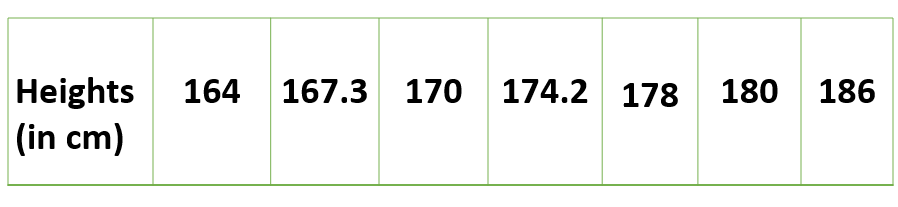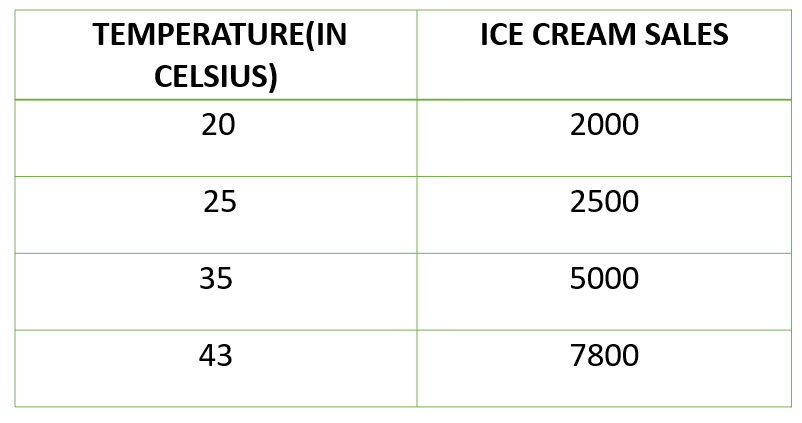Open In App

# Univariate, Bivariate and Multivariate data and its analysis

### 1. Univariate data –

This type of data consists of only one variable. The analysis of univariate data is thus the simplest form of analysis since the information deals with only one quantity that changes. It does not deal with causes or relationships and the main purpose of the analysis is to describe the data and find patterns that exist within it. The example of a univariate data can be height.Suppose that the heights of seven students of a class is recorded(figure 1),there is only one variable that is height and it is not dealing with any cause or relationship. The description of patterns found in this type of data can be made by drawing conclusions using central tendency measures (mean, median and mode), dispersion or spread of data (range, minimum, maximum, quartiles, variance and standard deviation) and by using frequency distribution tables, histograms, pie charts, frequency polygon and bar charts.

### 2. Bivariate data

This type of data involves two different variables. The analysis of this type of data deals with causes and relationships and the analysis is done to find out the relationship among the two variables. Example of bivariate data can be temperature and ice cream sales in summer season.Suppose the temperature and ice cream sales are the two variables of a bivariate data(figure 2). Here, the relationship is visible from the table that temperature and sales are directly proportional to each other and thus related because as the temperature increases, the sales also increase. Thus bivariate data analysis involves comparisons, relationships, causes and explanations. These variables are often plotted on X and Y axis on the graph for better understanding of data and one of these variables is independent while the other is dependent.

### 3. Multivariate data

When the data involves three or more variables, it is categorized under multivariate. Example of this type of data is suppose an advertiser wants to compare the popularity of four advertisements on a website, then their click rates could be measured for both men and women and relationships between variables can then be examined. It is similar to bivariate but contains more than one dependent variable. The ways to perform analysis on this data depends on the goals to be achieved. Some of the techniques are regression analysis, path analysis, factor analysis and multivariate analysis of variance (MANOVA).

There are a lots of different tools, techniques and methods that can be used to conduct your analysis. You could use software libraries, visualization tools and statistic testing methods. However, this blog we will be compare Univariate, Bivariate and Multivariate analysis.